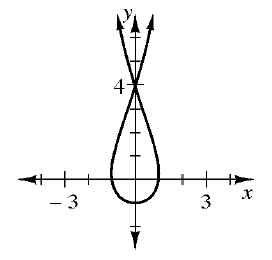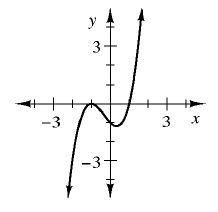### Home > CCA > Chapter 1 > Lesson 1.2.5 > Problem1-78

1-78.

Which of the relationships below are functions? If a relationship is not a function, give a reason to support your conclusion.

1.• There is more than one output value for some inputs; therefore, the relation is not a function.

1. $x$

$y$

$-3$

$19$

$5$

$19$

$19$

$0$

$0$

$-3$

• It is a function. Every input had only one output even if it is the same output such as $19$.

1.  $x$ $y$ $7$ $-2$ $0$ $7$ $4$ $10$ $0$ $10$ $3$ $0$
• There is more than one output value for $x=7$.
Is this relation a function?

1.• It is a function.